Back to School: Electricity Basics and how they apply to your Vehicles electrical system.

After my posting on the UBB I received a few emails with good suggestions for topics in future articles. I received one email in particular that was really informative. Here is the note I received from David Smith- the text in blue is my response to Davids thoughts.

OK class today's subject is "electricity"!

I think we have all had some electrical theory at some point or another- I know I had it in high school physics. In this article we'll talk about some electrical basic and apply them to audio systems and automotive electrical systems. We'll start off with the basics then move on to some more advanced stuff to see how it relates to speaker installs and amplifier loading.

FIGURE 1: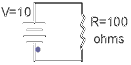This is a very simple electrical circuit. We have a source of electrical power, a path for the "electricity" to flow and a load that uses the electrical energy. OK lets delve a little deeper into this simple circuit and see what is going on.
Consider first the "supply" of the electricity. We can see that the above circuit has a supply voltage of 10 Volts- but what does this really mean? Well, for each volt (V) there is difference of  1 coulomb (6,280,000,000,000,000,000 electrons) from the negative terminal to the positive- so in the above example there are 10x 6,280,000,000,000,000,000 more electrons on the negative terminal than the positive. This potential difference is called the electromotive force (emf) and it's unit of measurement is the volt. The more volts the bigger the emf and more electrons to flow in the circuit and perform work for us. Once 1 coulomb of electrons has moved from the negative to the positive terminal the potential difference will only be 9 volts and once another coulomb has moved it will be only 8 volts and so on. It is for this reason batteries "die"- the number of electrons that have moved from the negative terminal to the positive is too many to perform anymore useful work. It is for this reason the automotive electrical system uses an alternator. The alternator charges the cars battery to keep the potential difference between the negative and positive terminal equal to 12 coulombs of electrons or 12V.
Now we are really going to confuse you! It's easier to think of electrical circuits when the electricity moves from positive to negative. However, we have already shown that electrons move from negative to positive so what gives?  Well, we know have to get into the atomic level of our circuit! If you remember back to high school physics each atom of a substance has a number of  electrons orbiting the nucleus. If the atom loses an electron there exists a "hole" that can be filled by another electron. The following diagram might clarify somewhat.

FIGURE 2: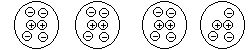.  As each electron moves from the left to the right to fill an open "hole" a new hole is created.  So the "holes" appear to move from right to left. Since an absence of an electron dictates a positive charge then the holes can be thought of as positive charges. Now it should be apparent that if the electrons move from negative to positive and the holes move opposite to the electrons, and are positive, then positive charges move from positive to negative. Clear as mud right?????From now on we will be assuming that the flow is from + to -. So if you look at the first diagram above the flow shown is for electrons and is opposite from what we will assume from now on.

In order for the "holes" to move from the positive plate to the negative they  must travel through a path that will conduct and not impede their journey. Gold makes an excellent conductor because it has many orbiting electrons that are available to move from one atom to the next. As we know though Gold is very expensive and as a result is impractical to use for circuitry. Copper, on the other hand, is relatively cheap and is also an excellent conductor-which explains why the majority of wire is made from copper.
In figure 1 you can see that the path to the load (the resistor) and back to the source appears to be made by wire connection. i.e. a wire is used to the load and another used back to the source. In a vehicle this would be very impractical as you would need a wire to each load and back to the battery. The load could be anything- turn signals, starter motor, rear window defogger, radio etc. Imagine the amount of wire needed! You would have twice as many wires in your car as you have now. So how did the designers get around this? Rather simply actually! The return path from the load to the battery is the metal of the car itself. So all the wire that would have been required is reduced to one huge "wire". Now by doing this you eliminate a ton of wire but you also introduce a bunch of problems. The worst of which is a ground loop and these play havoc with the audio signals in your stereo system. We'll talk much more about these problems in other articles. The important thing to get out of this section is the fact that the automobile uses a common return path. Figure 3 shows the interpretation of a common return circuit. It still functions exactly the same way as the circuit in figure 1.

FIGURE 3: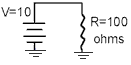Let's go back a little in order to discuss current. We talked about Coulombs of electrons moving from the negative to the positive plate- well, when the intensity of electrons flowing is such that when 1 Coulomb passes a given point in 1 second the electrical current flowing is 1 Ampere. The symbol for the current is (I) for intensity and the unit of measurement is the Ampere. So, 1 Ampere = 1 Coulomb / 1 second.
Remember we said that the "holes" flow opposite to the electron flow-therefore, 1 Ampere= 1 Coulomb(of holes) / 1 second.

Oh what the heck...here is the chart anyway...

Length of Run (in feet)

 Current 0-4 4-7 7-10 10-13 13-16 16-19 19-22 22-28 0-20A 14 12 12 10 10 8 8 8 20-35A 12 10 8 8 6 6 6 4 35-50A 10 8 8 6 6 4 4 4 50-65A 8 8 6 4 4 4 4 2 65-85A 6 6 4 4 2 2 2 0 85-105A 6 6 4 2 2 2 2 0 105-125A 4 4 4 2 2 0 0 0 125-150A 2 2 2 2 0 0 0 0

OK, we've talked about voltage, current and resistance. We can now combine that knowledge into a basic electrical law known as Ohm's Law. There exists a relationship between all three properties and knowing any two of them we can apply simple math to figure out the third. So, Ohms Law in a nutshell is   V=IxR. I.e the voltage drop across a load is equal to the current flowing through it multiplied by the resistance of the load itself.
Of course this can also be expressed as I=V/R and R=V/I
Using figure 3 as an example we can figure out the current flowing in the circuit..We know V=10volts and R=100 Ohms therefore; I=10/100 or I=0.1 Amps. This is more often than not expressed as 100mA (100 milli-Amps)
Each load will dissipate a certain amount of power and this too can be easily figured out  using another simple formula.
P=VxI and the unit of measurement is the Watt. Once again using figure 3 we can deduce the power dissipated is;
P=10x0.1 = 1watt    Once again, the formulas can be manipulated mathematically such that P= (VxV)/R=(IxI)xR.
Where is this of use to use? Well lets assume you have some fog lights that are rated at 100 Watts and want to know how much current it will require from the battery. You can easily figure it with the knowledge we have just learnt. We can assume the voltage from the car battery will be about 12V and we know the power dissipated is 100W therefore; I=P/V=100/12=8.3 Amps. Therefore, you will likely need a 10Amp fuse to hook up the lights.
One thing I should mention now is the fact that we are dealing with direct current (DC) analysis at the moment. Things change somewhat when we work with Alternating Current (AC). Remember those sine waves from article four? Well they are AC in nature so we will have to change our method of thinking shortly when we start working with resistance's offered by speakers etc.

Wiring Configurations:

Note all the diagrams were borrowed from :
http://members.xoom.com/jimas/ser_par.html

The circuit in figure 1 is know as a series configuration because there is only one path for the current to flow. Here is another circuit that is wired in series. The primary thing to remember is that the current value is constant in a series circuit.

figure 4: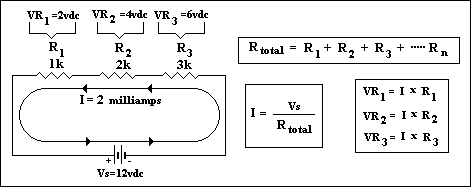Solving unknown values in any circuit really boils down to working with a) what values you do know b) It's properties (serries, parallel, combination) c) basic mathematical formulas
Consider the circuit to the left. We know the voltage and individual resistance values, we know it is a series circuit and we know some basic formulas so, with some thinking we can solve other "unknowns" about this circuit. In this example the current is given but you can easily find it if it wasn't by using ohms law. In a series circuit the total resistance is the sum of the individual ones. In this case it's 1K+2K+3K or 1000+2000+3000 i.e. 6000 (6K) Ohms. Now using Ohms law we can calculate the current by dividing the supply voltage by the total resistance. In this case it works out to 0.002Amps or 2 milliamps. Now we know one important fact- the current flowing in this Series circuit is 2milliamps. Why is it important? Remember  the property of a series circuit- current is constant, therefore that 2milliamps has to flow through each resistor. Now we can apply ohms law once again, to each resistor, to calculate the voltage drop accross it.  Applying the formula for power (P=VxI, and it's derivatives) we can calculate both the power dissipated in the individual resistors and the total power dissipated.

Series circuits are not widely used in the automotive environment because if the circuit breaks anywhere or a component opens then the circuit is dead as no current can flow. An example would be the old strings of Christmas tree lights- when a single bulb blew then the entire string was dead and it was a real pain trying to find the dead one. Parallel circuits are the most widely used configuration as there are multiple paths for current to flow. We'll look at them now.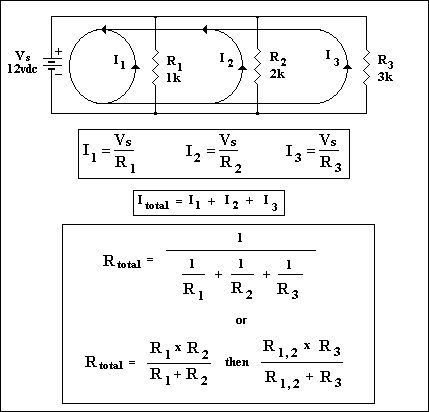This is a parallel configuration because there are multiple paths for current to flow. The main thing to remember about parallel circuits is that voltage is constant across each leg. However, Ohms law still applies and we can calculate unknown values. The hardest thing to calculate is the total resistance but the formulas shown in the diagram should help. You'll know that you are correct if the total resistance is smaller than the smallest resistance in the circuit. In this case the total resistance will be smaller than 1K Ohms. It works out to be 545.5 Ohms using the formula provided.
Using Ohms law again we can calculate the current flowing in each leg and then sum them all to find the total current demand on the supply.
OK let's figure that out...I1=12/1000=12mA, I2=12/2000=6mA, I3=12/3000=4mA and the total current is I1+I2+I3=22mA Using the power formulas we can calculate the power dissipated by each resistor and then sum the individual values to find the total. In this example P1=VxI1=12x0.012=0.144W, P2=VxI2=12x0.006=0.072W, P3=VxI3=12x0.004=0.048W therefore Ptotal=P1+P2+P3=0.264W. We could have also found Ptotal by using Pt=VxItotal=12x0.022=0.264W

In the automotive world nearly everything is wired in parallel. Each resistor, in the diagram, would be a load of some kind-maybe a tail light or starter motor etc.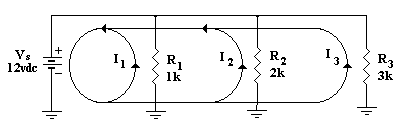I've redrawn the circuit to show the common return as it would appear in automotive diagrams. The calculations are exactly the same however. Ignore the arrows on the direction of current flow as it shows ELECTRON flow.
e last configuration we'll look at is the series-parallel circuit-which is just a     Thcombination of the two we've already looked at. The trick is to use the knowledge we've learned so far and take bits and pieces to try and solve unknown values.
Consider this circuit.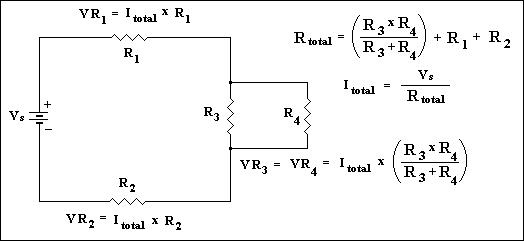As you can see part of the circuit appears to be parallel and part series. Ohms law STILL works! You can find voltage, current and power through each resistor if you think logically and use the formulas we know. We're  not going to solve  the values in this example but we'll discuss a logical path to take to go about solving the values. First combine R3 and R4 in to one value known as R34 using the formulas given in the parallel circuits. Now you could redraw the circuit as a simple series circuit replacing R3 and R4 with a single calculated value R34. You'll notice you now have a simple series circuit. What do we know about Series circuits? Current is Constant! It might be an idea to find this value. Simply add all resistor values to find Rtotal =R1+R2+R34 and divide this into Vs to get Itotal. Now you can use Ohms law to find the voltage drop across R1 and R2 and using by using; P=VxI find the power dissipated. Now we have to solve the values for the section we simplified i.e. R3 and R4. Redraw the original circuit and fill in all the values we know already. We know everything except the values for the parallel section. What do we know about parallel circuits? Voltage is constant! We know the voltage drop across R1 and R2 and we know the total voltage supplied therefore to find the voltage across the parallel circuit section simply subtract (Vr1+Vr2) from Vs (Vs=Vr1+Vr2+Vr34). Now we can use Ohms law again to find the current flowing  through R3 and R4. We now know the voltage so it's just a matter of dividing this voltage by each resistor value for R3 and R4. Power can now be calculated using any of the power formulas.
There! As you can see it is not that difficult to use Ohms law. You just have to break the circuit down into a simpler parallel or series circuit and solve for values which can be used to find other unknown values when you look at the original circuit. OK Now lets look at how this all applies to audio systems.

Ohm's Law and Audio Systems:

You would most likely use what we've learned so far to calculate the speaker impedance to an amplifier channel. There are some things we should probably discuss before we get too far into this portion of our article. If you recall from article 4    we saw that it was the speakers voice coil that was connected to an amplifiers channel. A coil (as in "Voice coil") changes it's resistance with different frequencies. This "resistance" should actually be referd to as an impedance as resistance is usually a term used for pure resistive loads. Coils and capacitors are not pure resistive-their "resistance" changes with frequency. Another term you may hear is reactance. Inductive reactance is the impedance at a specific frequency for a coil and capacitive reactance is the impedance for a capacitor at a specific frequency. The unit of measurement for both is still the Ohm but instead of  'R' as the symbol for resistance we use 'Xl' for inductive reactance and 'Xc' for capacitive reactance. We don't need to worry too much about these reactances when determining amplifier load but they do play a major role when designing crossovers. We'll work on a few examples a bit later on just so you can get an idea of how frequency affects the impedance value.
OK back to load calculations.....When you buy a speaker it is normally given a nominal value for the D.C impedance. These values are normally either 4 or 8 Ohm but some are available in 6 Ohm values. Why the different choices? Well, the   configuration you use them in will play a role in your decision as to which Ohm value you should buy. This nominal value is about 1.33 times (EIA standards) the actual DC resistance of the speaker i.e. the approximate value you would read if you used an ohm meter to measure the resistance of the coil.
We hook speakers up to the amplifiers using circuit configurations we have already looked at namely either in 1) series 2) parallel or 3) series parallel. What does this mean then? Answer: OHM"S LAW STILL APPLIES!!!

Let's consider a practical example: Let's say you have a dedicated sub woofer amplifier to power the subs. It might have the following specifications- 50Wx2 into 4 Ohms, 125Wx1 into 4Ohms or 150Wx1 into 2 Ohms. How do you interpret this? If you use both channels to power speakers then you can get a total of 100W (50Wx2)power in to the speakers. However, if you were to combine both channels into one one bigger channel (known as bridging) then you can get more power output. We'll look at bridging in detail in later articles. What we want to concentrate on in this article is how to configure the speakers to get the most power output. In our fictitious amplifier the most power output is noticed when the speakers are configure to represent a total impedance of  2 Ohms. So the idea is to wire the speakers to get a total impedance of 2 Ohms. We'll use four different examples going from using one speaker  to two to three and finally to four.

One speaker:
It's rare that you will find a 2 Ohm speaker so in most cases you will only be able to get a 4 Ohm impedance which means your amp will put out 125 Watts into this single speaker. However, if your single speaker has DUAL voice coils then you can wire them as if you had two speakers and get a 2 Ohm load. This is accomplished by wiring the voice coils in parallel. (+ to + and - to -).
figuring out the final resistance is actually quite easy if all the speakers you are connecting together have the same impedance (4,6 or 8 Ohm).When connected in series (+ of one to  - of the other) you simply add the impedance's. So, two 4 ohm speakers in series is an 8 Ohm total impedance. Three in series is 12 Ohms etc. In a parallel configuration you take the impedance of one speaker and divide it by the total number of speakers you are connecting. Two 4 Ohm speakers connected in parallel will yield a total impedance of 2 Ohms (4/2 i.e. 4 ohms/2 speakers). Four 8 Ohm speakers in parallel is a 2 Ohm total impedance (8/4). This rule ONLY applies to combining speakers with the same impedance.

Two speakers:
With two speakers (or one dual voice coil speaker) we have a few options. If we want to use 4 Ohm speakers then when combined in parallel the amplifier will see a total impedance of 2 Ohms and the maximum power will be seen. Combined in series the total impedance will  be 8 Ohms and the power out put of the amp will be much less. With 8 Ohm speakers the total impedance will be 4 Ohms in parallel and 16 Ohms in series. As you can see  knowing at what impedance the maximum power is realized and then combining the correct impedance speaker(s) can have a huge affect on the power output from the amplifier. Now if you used two dual coil speakers you would try and configure them to get a 2 ohm total impedance to maximize the power. We'll save this configuration until we look at combining 4 speakers together as it will be the same.

Three Speakers:
Combining three speakers together is normally not a good combination with 4 ohm speakers as the impedance will be too low if connected in parallel or too high if connected in series. With 8 ohm speakers you could hook them in parallel and end up with a total impedance slightly higher than 2 ohms (8/3 to be exact). Now you could combine two 8 ohm speakers in parallel then put them in parallel with a 4 ohm speaker to get the ideal 2 ohm load. However, this is not recommended because the 4 ohm speaker will twice as much power as the parallel combination of  the 8 ohm speakers.
There are some manufacturers that now offer 6 ohm speakers so that when you use 3 of them in parallel you will get the ideal 2 ohm load.

Four speakers: (or two dual voice coil speakers)
With 8 ohm speakers you can hook them all in parallel and get a 2 ohm load (8/4=2). with 4 ohm speakers the best we can hope for is a total load of 4 ohms. To accomplish this we hook two speakers that are wired in series with each other in parallel with another two speakers that are also wired in series with each other. Mathematically we can simplify the "circuit" by assuming we now have two 8 ohm speakers in parallel with each other. Therefore we have (8/2=4) ohm total.

As you can see Ohms law still works with speakers if you use the nominal speaker impedance in the calculations i.e. 4,6 or 8 ohm (in general). However, once the frequency of the music is introduced the overall impedance to an amplifier can change significantly. We'll have a look at this briefly now. It will be covered in more detail in later articles when we look at crossovers.

Frequency and Impedance:

One of the speakers main components is the voice coil. The DC value of this coil is what is used to give us the nominal speaker impedance. The nominal impedance is about 1.33 times the actual DC value. So, a 4 Ohm speaker is really 4/1.33 Ohms.
Once the speaker starts to play music though the impedance value will change depending on the frequency at that instance in time.
The impedance at that frequency is called the inductive reactance (Xl) and is calculated using this formula
Xl=2(pi)FL where pi is 3.14blah blah blah, F is frequency and L is the inductance value of the coil.

L and pi are constant and do not change therefore the variables are Xl and F. We can see that if the frequency increases then the inductive reactance increases also. When the frequency decreases then the inductive reactance also decreases.